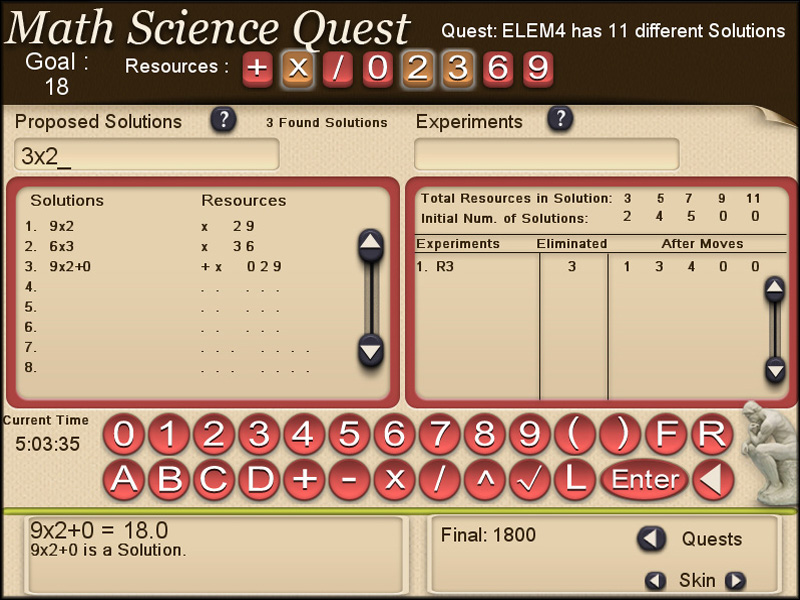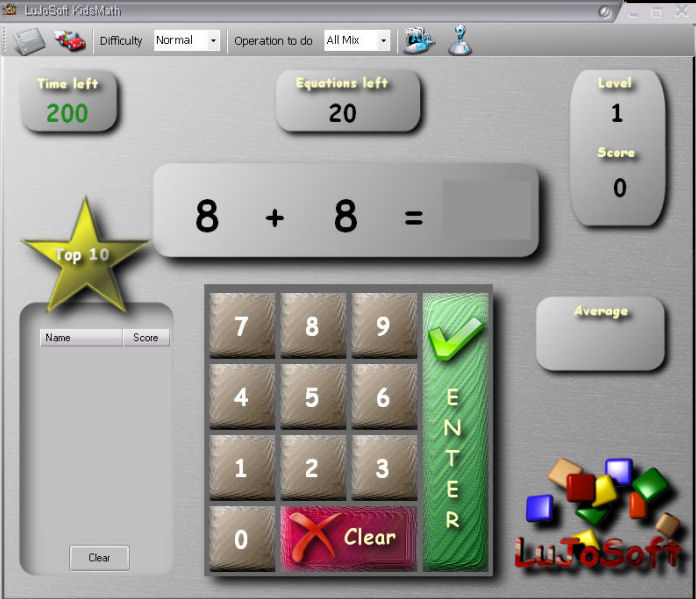# N5 Net Software Repository

Search Files

Only Freeware

### Authors Area

Are you a software author? Take a look at our Author Resource Center where you will find marketing tools, software specifically created for promoting your software and a ton of other helpful resources.

### ACASTAT WIN 10.5.2

Education :: MathematicsAcaStat statistical software is an inexpensive and easy-to-use data analysis tool. Create and analyze crosstabulations, descriptive statistics, basic significance tests, and summary reports. Ideal for those who need a low-cost alternative for producing basic summary statistics at school or work. Create data files or import from spreadsheets or statistical software. Practice data files. Integrated update module. Available in Mac and Windows.
Windows | Demo

### STATCALC WIN 9.1.6

Education :: MathematicsThis user-friendly analytical tool simplifies common statistical calculations with 31 procedures. Use StatCalc to easily create and compare summary statistics, develop confidence intervals, and verify hand calculations. Enter data to create descriptive, correlation, and regression statistics. A Decision Tools module is included to explore price elasticity of demand, queuing theory, constant dollars, and decision tables. Mac and Windows.
Windows | Demo

### GRAPHMATICA 2.4

Education :: Mathematicsa powerful, easy-to-use, equation plotter with numerical and calculus features. Graph Cartesian functions, relations, and inequalities, plus polar, parametric, and ordinary differential equations. Numerically solve and graphically display tangent lines and integrals. Find critical points, solutions to equations, and intersections between Cartesian functions. For students and teachers from high-school algebra through college calculus.
Windows | Shareware

### EQUATION GRAPH PLOTTER - EQPLOT 1.3.57

Education :: MathematicsEqPlot plots 2D graphs from complex equations. The application comprises algebraic, trigonometric, hyperbolic and transcendental functions. EqPlot can be used to verify the results of nonlinear regression analysis program. Graphically Review Equations: Up to ten equations could be plotted at the same time, so that intersections and domains could be studied visually.
Windows | Shareware

### CALCULATIONLABORATORY 1.4.19.1540

Education :: MathematicsCalculationLaboratory - mathematical program that can handle scripts (their own programming language), and also can handle scripts extreme number (decimal). There is support for multi-threading (using potentiation, ie exponentiation). There is also the Builder of the graphs. You may plotting to use both conventional and extreme number. Supports working with databases for better data cooperation.
Windows | Shareware

### MATH SCIENCE QUEST 1.4

Education :: MathematicsMath Science Quest is a fun way to learn the fundamental reasoning skills that are the essence of scientific method. It can be played as an individual puzzle or a multi-player game. Players explore a complex mathematical puzzle attempting to find all the solutions. Experiments can be designed to provide additional information about the resources and concepts used in the undiscovered solutions.
Windows | Freeware

### KIDSMATH 9.0.3.1

Education :: MathematicsIs a game that teach math table 0 to 12 for children ages 5 to 9. What better way to learn that playing a game. Feature in KidsMath: Every level consists of 20 questions. KidsMath as 4 different difficulty level that affect the play time. Easy 300 seconds. Normal 200 seconds.
Windows | Freeware

### SIMPLELPSOLVER 1.3.1A

Education :: MathematicsThe Simple LP Solver can solve large size linear programming problems in an efficient way. The size of the problem theoratically depends on the memory allocated to the program by the computer; practically total array size could be max. 128M. The program provides Simplex and Revised Simplex solvers. If the all constraints are <=, the single phase technique is applied while Two Phased technique is automatically used for others; = and >=
Windows | FreewareFree Learn Addition contains 8 lessons on basic addition and you learn them on board the Orbital One space station. To ensure you have grasped the subject 27 tests have been included. Free Learn Addition is the addition topic of Learn Math 3D on Orbital One made in to a fully functional stand alone program. A large HTML help file and easy to use controls should help the student get up to speed quickly.